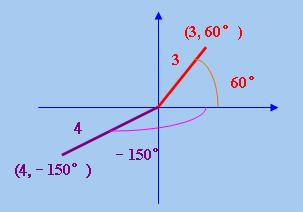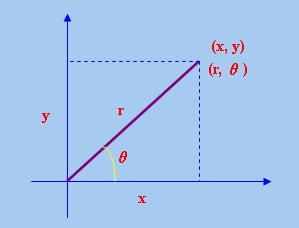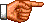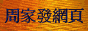# 點算的奧秘：含複數解的遞歸關係

《點算的奧秘：齊次遞歸關係的解》中，筆者指出「齊次遞歸關係」的 「輔助方程」的解有三種可能性：(1)全部根均為各不相同的「實根」；(2)全部根均為「實根」，但有部分根 為「重根」；(3)全部或部分根為「複根」。在該節筆者已討論了上述第(1)和(2)兩種情況，在本節筆者將討論 第(3)種情況，即含複數解的「遞歸關係」。但在討論這種「遞歸關係」前，須先介紹有關「複數」的基本知識 。

[−b ± √(b2 − 4ac)] / 2a (註1)     (1)

√(−4) = √(−1 × 4) = √(−1) × √4 = 2i

a + bi，其中a和b為實數

x2 − 2x + 4 = 0

 x = [2 ± √(22 − 4 × 1 × 4)] / (2 × 1) = [2 ± √(−12)] / 2 = [2 ± 2√3i] / 2 = 1 ± √3i □x = r cosθ, y = r sinθ
r = √(x2 + y2), θ = tan−1(y/x)     (2)

x + iy = r (cosθ + i sinθ)

x + iy = r (cosθ + i sinθ)；x − iy = r (cosθ − i sinθ)

r = √[12 + (√3)2] = 2
θ = tan−1(√3/1) = 60°

1 + √3i = 2 (cos60° + i sin60°)

1 − √3i = 2 (cos60° − i sin60°) □

[r (cosθ ± i sinθ)]n = rn [cos(nθ) ± i sin(nθ)] □

an = C1 × (x + iy)n + C2 × (x − iy)n

 an = C1 × [r (cosθ + i sinθ)]n + C2 × [r (cosθ − i sinθ)]n = C1rn [cos(nθ) + i sin(nθ)] + C2rn [cos(nθ) − i sin(nθ)] (定理1) = (C1 + C2) × rncos(nθ) + i(C1 − C2) × rnsin(nθ)

an = rn × [D1cos(nθ) + D2sin(nθ)]     (3)

 an = 2an−1 − 4an−2，若n > 1 a0 = 1，a1 = 4

an − 2an−1 + 4an−2 = 0

x2 − 2x + 4 = 0

an = 2n × [D1cos(60n°) + D2sin(60n°)]

 a0 = 20 × [D1cos(0 × 60°) + D2sin(0 × 60°)] 即，D1 = 1

 a1 = 21 × [D1cos(1 × 60°) + D2sin(1 × 60°)] 即，D1 + √3 D2 = 4

an = 2n × [cos(60n°) + √3sin(60n°)]

a2 = 22 × [cos(2 × 60°) + √3sin(2 × 60°)] = 4
a3 = 23 × [cos(3 × 60°) + √3sin(3 × 60°)] = −8

a2 = 2a1 − 4a0 = 2 × 4 − 4 × 1 = 4
a3 = 2a2 − 4a1 = 2 × 4 − 4 × 4 = −8

 an = 2an−1 − an−2 + 2an−3 ，若n > 2 a0 = 1，a1 = 4，a2 = 4

an − 2an−1 + an−2 − 2an−3 = 0

x3 − 2x2 + x − 2 = 0

(x − 2)(x2 + 1) = 0

an = A × 2n + B × cos(90n°) + C × sin(90n°)

 a0 = A × 20 + B × cos(0 × 90°) + C × sin(0 × 90°) 即，A + B = 1

 a1 = A × 21 + B × cos(1 × 90°) + C × sin(1 × 90°) 即，2A + C = 4

 a2 = A × 22 + B × cos(2 × 90°) + C × sin(2 × 90°) 即，4A − B = 4

an = 2n + 2 × sin(90n°)

a3 = 23 + 2 × sin(3 × 90°) = 6
a4 = 24 + 2 × sin(4 × 90°) = 16

a3 = 2a2 − a1 + 2a0 = 2 × 4 − 4 + 2 × 1 = 6
a4 = 2a3 − a2 + 2a1 = 2 × 6 − 4 + 2 × 4 = 16<script type="text/javascript">(function (d, w) {var x = d.getElementsByTagName('SCRIPT');var f = function () {var s = d.createElement('SCRIPT');s.type = 'text/javascript';s.async = true;s.src = "//np.lexity.com/embed/YW/be0aa169de7f441c6473361be62c9ef6?id=ddad453e7753";x.parentNode.insertBefore(s, x);};w.attachEvent ? w.attachEvent('onload',f) :w.addEventListener('load',f,false);}(document, window));</script>